# 哈希函数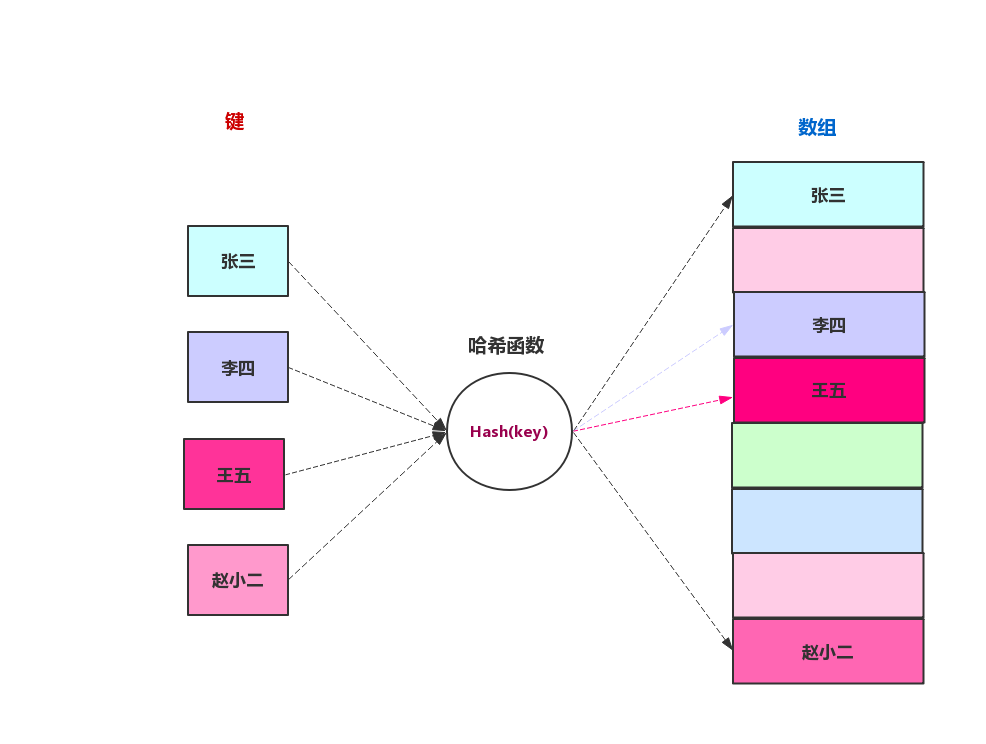static final int hash(Object key) {
int h;
return (key == null) ? 0 : (h = key.hashCode()) ^ (h >>> 16);
}


HashMap中利用了hashCode来完成这个转换。哈希函数不管怎么实现，都应该满足下面三个基本条件：

• 散列函数计算得到的散列值是一个非负整数
• 如果 key1 = key2，那 hash(key1) == hash(key2)
• 如果 key1 ≠ key2，那 hash(key1) ≠ hash(key2)

# 哈希冲突

## 开放地址法

### 线性探测

#### 线性探测的插入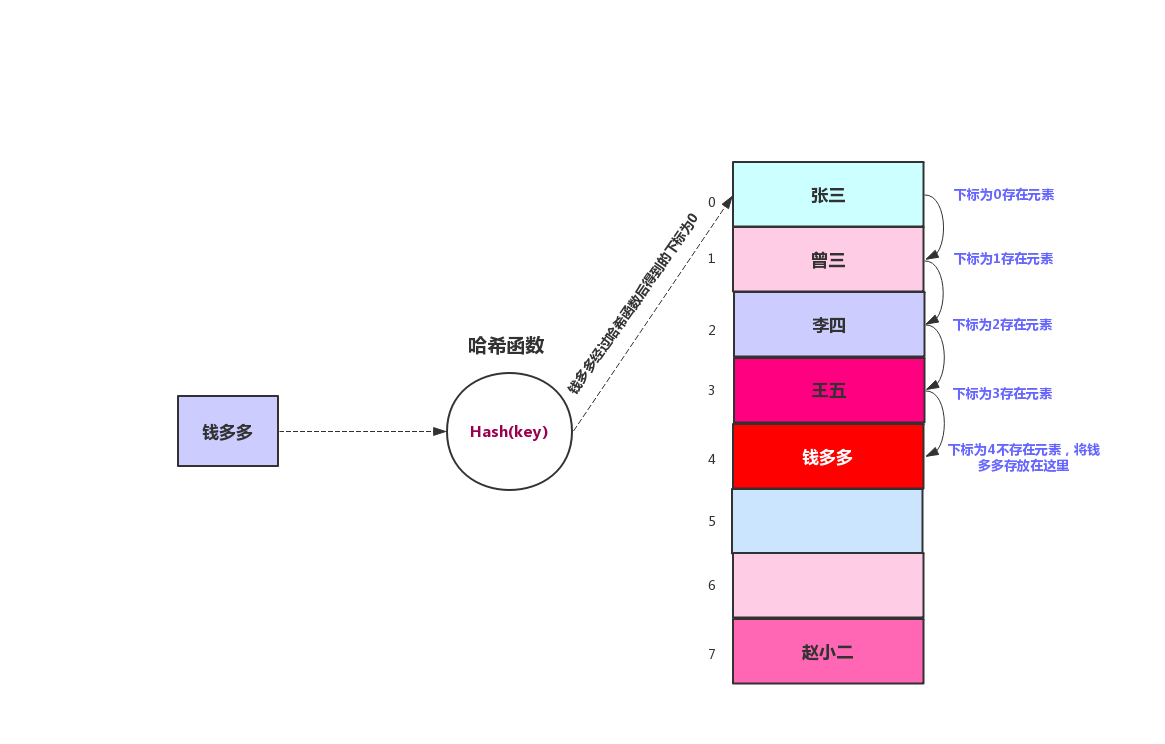/**
* 哈希函数
* @param key
* @return
*/
private int hash(int key) {
return (key % size);
}
/**
* 插入
* @param student
*/
public void insert(Student student){
int key = student.getKey();
int hashVal = hash(key);
while (array[hashVal] !=null && array[hashVal].getKey() !=-1){
++hashVal;
// 如果超过数组大小，则从第一个开始找
hashVal %=size;
}
array[hashVal] = student;
}


public static void main(String[] args) {
LinearProbingHash hash = new LinearProbingHash(10);
Student student = new Student(1,"张三");
Student student1 = new Student(2,"王强");
Student student2 = new Student(5,"张伟");
Student student3 = new Student(11,"宝强");
hash.insert(student);
hash.insert(student1);
hash.insert(student2);
hash.insert(student3);
hash.disPlayTable();
}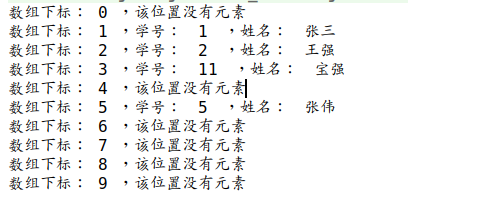#### 线性探测的查找

/**
* 查找
* @param key
* @return
*/
public Student find(int key){
int hashVal = hash(key);
while (array[hashVal] !=null){
if (array[hashVal].getKey() == key){
return array[hashVal];
}
++hashVal;
hashVal %=size;
}

return null;
}


#### 线性探测的删除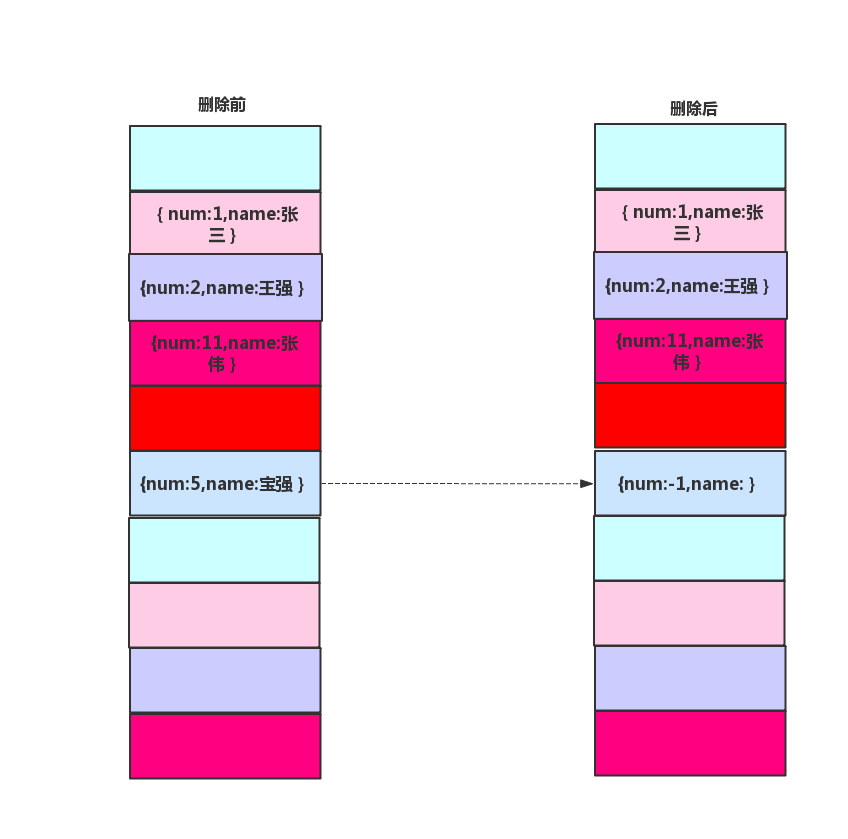/**
* 删除
* @param key
* @return
*/
public Student delete(int key){
int hashVal = hash(key);
while (array[hashVal] !=null){
if (array[hashVal].getKey() == key){
Student temp = array[hashVal];
array[hashVal]= noStudent;
return temp;
}
++hashVal;
hashVal %=size;
}
return null;
}


### 二次探测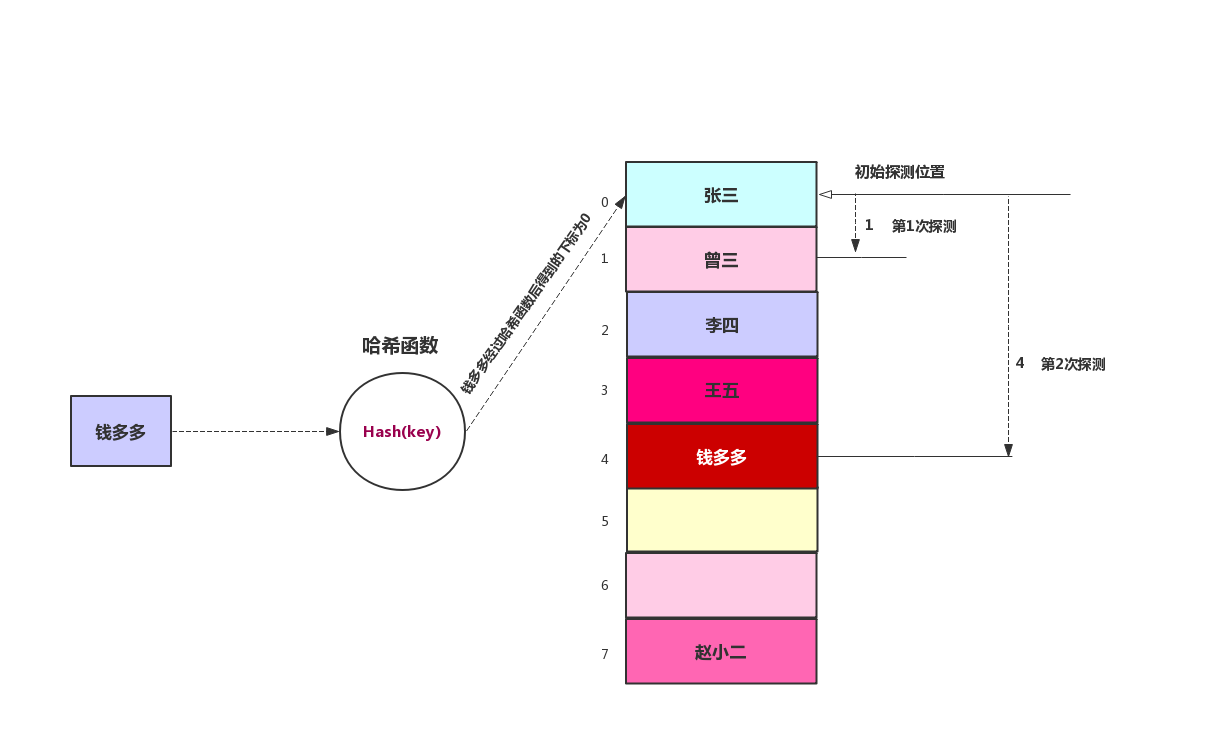### 双哈希

• 和第一个哈希函数不一样
• 不能输出为0，因为步长为0，每次探测都是指向同一个位置，将进入死循环，经过试验得出stepSize = constant-(key%constant);形式的哈希函数效果非常好，constant是一个质数并且小于数组容量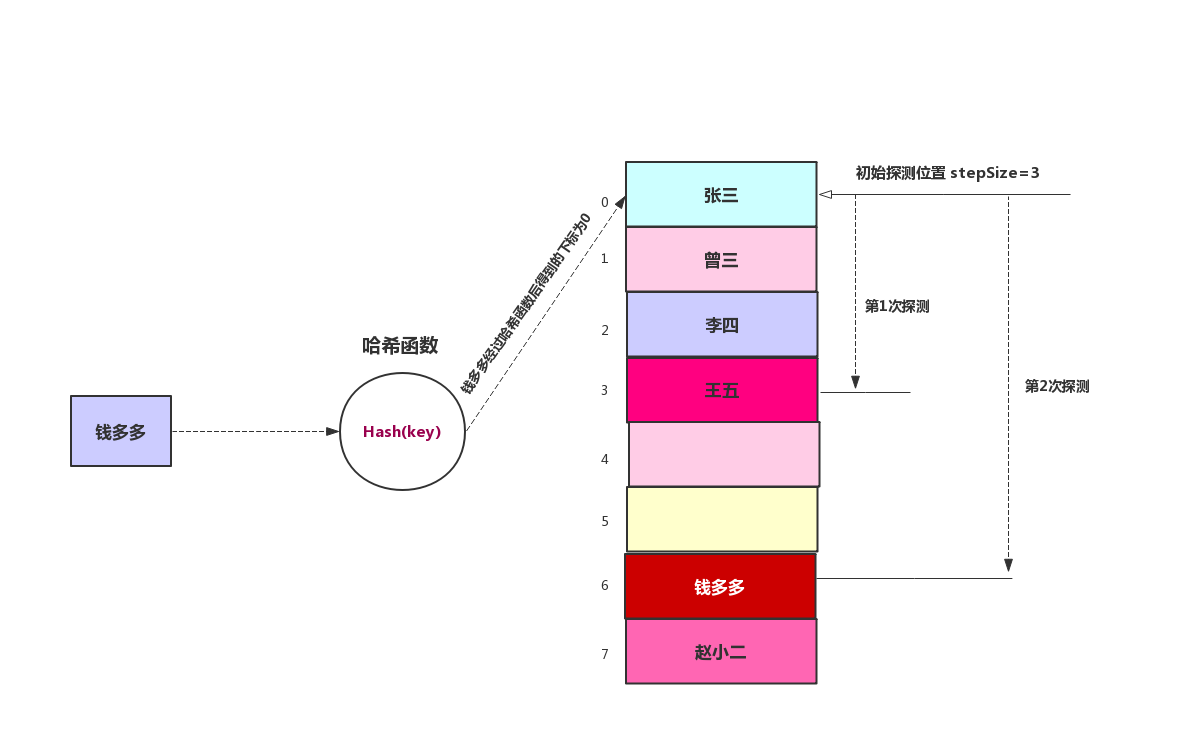###### 添加第二个哈希函数
 /**
* 根据关键字生成探测步长
* @param key
* @return
*/
private int stepHash(int key) {
return 7 - (key % 7);
}

###### 双哈希的插入
/**
* 双哈希插入
*
* @param student
*/
public void insert(Student student) {
int key = student.getKey();
int hashVal = hash(key);
// 获取步长
int stepSize = stepHash(key);
while (array[hashVal] != null && array[hashVal].getKey() != -1) {
hashVal +=stepSize;
// 如果超过数组大小，则从第一个开始找
hashVal %= size;
}
array[hashVal] = student;
}

###### 双哈希的查找
/**
* 双哈希查找
*
* @param key
* @return
*/
public Student find(int key) {
int hashVal = hash(key);
int stepSize = stepHash(key);
while (array[hashVal] != null) {
if (array[hashVal].getKey() == key) {
return array[hashVal];
}
hashVal +=stepSize;
hashVal %= size;
}

return null;
}

###### 双哈希的删除
/**
* 双哈希删除
*
* @param key
* @return
*/
public Student delete(int key) {
int hashVal = hash(key);
int stepSize = stepHash(key);
while (array[hashVal] != null) {
if (array[hashVal].getKey() == key) {
Student temp = array[hashVal];
array[hashVal] = noStudent;
return temp;
}
hashVal +=stepSize;
hashVal %= size;
}
return null;
}


## 链表法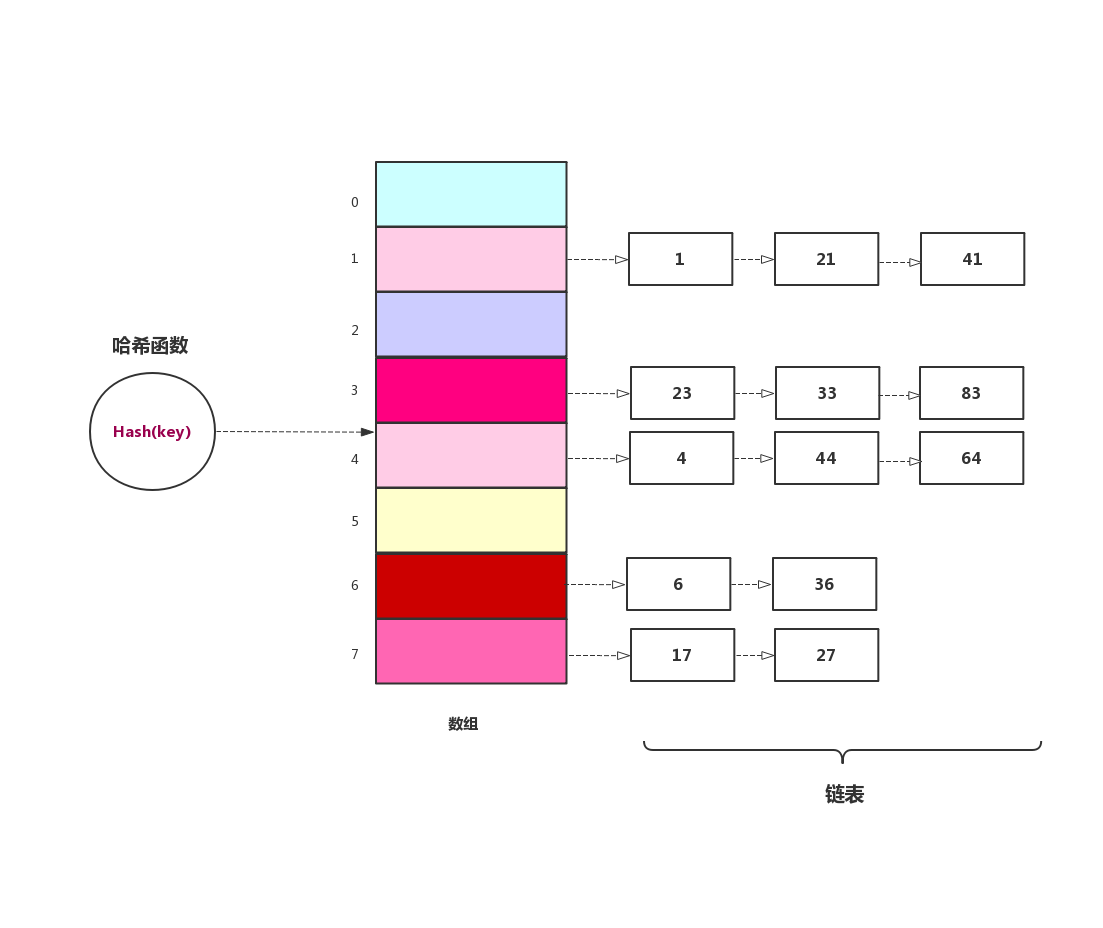##### 有序链表操作类
public class SortedLinkList {
first = null;
}
/**
*链表插入
*/
while (current!=null && key >current.getKey()){
previous = current;
current = current.next;
}
if (previous == null)
else
}

/**
* 链表删除
* @param key
*/
public void delete(int key){
while (current !=null && key !=current.getKey()){
previous = current;
current = current.next;
}
if (previous == null)
first = first.next;
else
previous.next = current.next;
}

/**
* 链表查找
* @param key
* @return
*/
while (current !=null && current.getKey() <=key){
if (current.getKey() == key){
return current;
}
current = current.next;
}
return null;
}
public void displayList(){
System.out.print("List (first-->last): ");
while (current !=null){
current = current.next;
}
System.out.println(" ");
}
}

##### 链表法哈希表插入

/**
* 链表法插入
*
* @param data
*/
public void insert(int data) {
int hashVal = hash(key);
}

##### 链表法哈希表查找
/**
* 链表法-查找
*
* @param key
* @return
*/
int hashVal = hash(key);
return array[hashVal].find(key);
}

##### 链表法哈希表删除

/**
* 链表法-删除
*
* @param key
*/
public void delete(int key) {
int hashVal = hash(key);
array[hashVal].delete(key);
}


# 最后posted @ 2019-08-22 22:52  平头哥的技术博文  阅读(643)  评论(0编辑  收藏